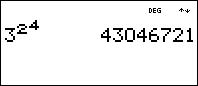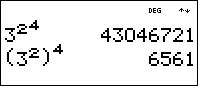# Knowledge Base

## Solution 20529: Incorrect Answer Received When Computing Exponents in MathPrint Mode Using the TI-30XS/B or TI-34 MultiView™ Scientific Calculator.

### Why do I receive an incorrect answer when I compute some problems in MathPrint mode with exponents using the TI-30XS/B or TI-34 MultiView calculator?

The TI-30XS/B and TI-34 MultiView calculator in MathPrint mode uses the Equation Operating System (EOS). EOS defines the order in which functions in expressions are entered and evaluated on the calculator. EOS lets the user enter numbers and functions in a simple, straightforward sequence. Within a priority level, EOS evaluates from left to right. Calculations within parentheses are evaluated first. This means that parentheses must be used in order to define the parts.

Example: 324Solution:

• Press [ ( ] and input 3.
• Press [x2] [ ) ].
• Press [^] and input 4. Press the [] key once.
• Press [enter].